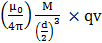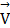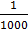# Two magnetic dipoles X and Y are placed at a separation d, with their axes perpendicular to each other. The dipole moment of Y is twice that of X. A particle of charge q is passing through their mid-point P, at angle= 45° with the horizontal line, as shown in figure. What would be the magnitude of force on the particle at that instant? (d is much larger than the dimensions of the dipole)(a) 0 (b)(c)(d)## Question ID - 51176 :- Two magnetic dipoles X and Y are placed at a separation d, with their axes perpendicular to each other. The dipole moment of Y is twice that of X. A particle of charge q is passing through their mid-point P, at angle= 45° with the horizontal line, as shown in figure. What would be the magnitude of force on the particle at that instant? (d is much larger than the dimensions of the dipole)(a) 0 (b)(c)(d)3537Since M y = 2Mxnet is parallel to= 0

Next Question :
 A damped harmonic oscillator has a frequency of 5 oscillations per second. The amplitude drops to half its value for every 10 oscillations.  The time it will take to drop toof the original amplitude is close to (a) 100 s (b) 10 s (c) 50 s (d) 20 s